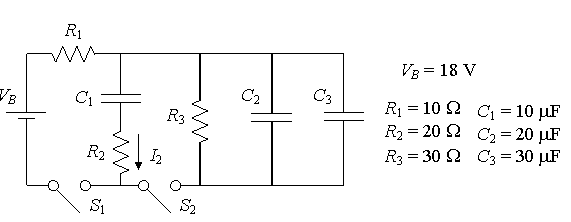# Three capacitors RC circuit questions

## Homework Statement

Three resistors, three capacitors, a battery and two switches are connected in the circuit shown below. The values of all circuit elements are given in the figure. Originally, the switches S1 and S2 are open (as shown) and all of the capacitors are uncharged. At time t = 0, both switches are closed.

http://i.imgur.com/BOq2c.pngPart A

What is the charge Q2 on capacitor C2 a very long time after the switches are closed?
(a) Q2 = 0 μC
(b) Q2 = 33 μC
(c) Q2 = 90 μC
(d) Q2 = 180 μC
(e) Q2 = 270 μC (Correct Answer)

Part B

After a very long time with both switches in the closed position, switch S2 is reopened. How long (t1/e) does it take for the charge on capacitor C2 to drop to 1/e (36.8%) of its fully-charged value (i.e. of the value it had just before S2 was reopened)?
(a) t1/e = 1200 μsec
(b) t1/e = 1500 μsec (Correct Answer)
(c) t1/e = 3000 μsec
(d) t1/e = 3600 μsec
(e) t1/e = 4800 μsec

KVL,KCL

## The Attempt at a Solution

For part A, what I tried is
Voltage across is 18 X ¾ (R3/ (R1+R3))= 27/2
(Because Q=CV and in this case Ic=0 so no current on R2?)
I get right answer, just not sure if I am thinking correctly.

For part B,
I used

Q= Q(0)e^-(t/tau)

However, I am not sure how do I find time constant in this case.

THX!

gneill
Mentor
Your part A method is fine.

For part B, once switch S2 is opened the right hand portion of the circuit is isolated from the left hand portion. So it's just two parallel capacitors and a resistor. What does that suggest to you?

Your part A method is fine.

For part B, once switch S2 is opened the right hand portion of the circuit is isolated from the left hand portion. So it's just two parallel capacitors and a resistor. What does that suggest to you?

How about R1 and R3 in this case?
Are they in parallel?
Thanks

gneill
Mentor
How about R1 and R3 in this case?
Are they in parallel?
Thanks

No! With switch S2 open they are isolated from each other (no complete circuit).